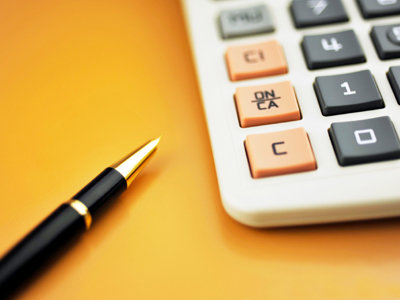# Exponents - Add and Subtract

This Math quiz is called 'Exponents - Add and Subtract' and it has been written by teachers to help you if you are studying the subject at middle school. Playing educational quizzes is a fabulous way to learn if you are in the 6th, 7th or 8th grade - aged 11 to 14.

It costs only \$12.50 per month to play this quiz and over 3,500 others that help you with your school work. You can subscribe on the page at Join Us

An exponent is a small superscript number that tells you how many times a number is multiplied by itself. For example, if you have the number 4 and you want to multiply it by itself five times you could write it out as follows:

4 x 4 x 4 x 4 x 4 =

This can become too long to always write out so we use a shortcut using a superscript number. In this case the superscript number would be “5” because we want to multiply 4 times itself five times. To show this using a superscript it will look like:

45

45 = 4 x 4 x 4 x 4 x 4

We then solve it as: 4 x 4 = 16 x 4 = 64 x 4 = 256 x 4 = 1024

45 = 1024

We can then add and/or subtract using exponents as follows:

32 + 73 =
3 x 3 = 9
7 x 7 = 49 x 7 = 343
9 + 343 = 352
32 + 73 = 352

1.
282 - 132 =
953
615
60
82
282 - 132 =
28 x 28 = 784
13 x 13 = 169
784 - 169 = 615
282 - 132 = 615
2.
122 + 75 =
16951
588
133
2545
122 + 75 =
12 x 12 = 144
7 x 7 = 49 x 7 = 343 x 7 = 2401 x 7 = 16807
144 + 16807 = 16951
122 + 75 = 16951
3.
10 + 44 - 43 =
202
330
70
14
10 + 44 - 43 =
4 x 4 = 16 x 4 = 64 x 4 = 256
4 x 4 = 16 x 4 = 64
10 + 256 = 266 - 64 = 202
10 + 44 - 43 = 202
4.
82 + 23 - 14 =
58
8
36
54
82 + 23 - 14 =
8 x 8 = 64
2 x 2 = 4 x 2 = 8
64 + 8 = 72 - 14 = 58
82 + 23 - 14 = 58
5.
1002 - 502 + 17 =
268
12517
117
7517
1002 - 502 + 17 =
100 x 100 = 10000
50 x 50 = 2500
10000 - 2500 = 7500 + 17 = 7517
1002 - 502 + 17 = 7517
6.
102 + 123 - 92 =
91
1909
1747
38
102 + 123 - 92 =
10 x 10 = 100
12 x 12 = 144 x 12 = 1728
9 x 9 = 81
100 + 1728 = 1828 - 81 = 1747
102 + 123 - 92 = 1747
7.
24 + 93 =
35
745
77
113
24 + 93 =
2 x 2 = 4 x 2 = 8 x 2 = 16
9 x 9 = 81 x 9 = 729
16 + 729 = 745
24 + 93 = 745
8.
73 + 34 + 16 =
408
49
35
440
73 + 34 + 16 =
7 x 7 = 49 x 7 = 343
3 x 3 = 9 x 3 = 27 x 3 = 81
343 + 81 + 16 = 440
73 + 34 + 16 = 440
9.
53 + 64 =
341
77
39
1421
53 + 64 =
5 x 5 = 25 x 5 = 125
6 x 6 = 36 x 6 = 216 x 6 = 1296
125 + 1296 = 1421
53 + 64 = 1421
10.
63 + 54 - 35 =
88
598
589
53
63 + 54 - 35 =
6 x 6 = 36 x 6 = 216
5 x 5 = 25 x 5 = 125 x 5 = 625
3 x 3 = 9 x 3 = 27 x 3 = 81 x 3 = 243
216 + 625 = 841 - 243= 598
63 + 54 - 35 = 598
Answer (b), therefore, shows the correct Order of Operation
Author:  Christine G. Broome